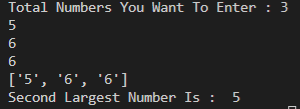# Python Find Second Largest Number In List

In this post , we will see how to find second maximum number in list

## Second Highest Number In List

### How To Find Second Largest Number In List Using Python

Firstly we need to ask user to enter total count of numbers they want to store. After that we will append them in a list.

#### Python Code To Find Second Maximum Number In Python

``````nums=[]
b=int(input("Total Numbers You Want To Enter : "))
for x in range(0,b):
en=input()
nums.append(en)
print(nums)
nums.sort()
final=set(nums)
highest=max(nums)
final.remove(highest)
print("Second Largest Number Is : ",max(final))``````Output
Using this code , we can find second largest number in a list using python. Note that it will also remove duplicate numbers so that it can find second largest number
.